Next: 4.5 Conclusions Up: 4. Spectral Properties of Previous: 4.3 Results

# 4.4 Discussion

For a tight-binding or a linear dispersion, the B polaron properties at small momentum are determined by smallscatterings characterized by small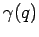. Let's consider the first order self-energy diagram contribution(4.27)

The most significant contribution below the one phonon threshold, i.e. when, is given by the values of transferred momentumfor whichis minimum which implies. The value ofwhere the polaron energy reaches the one-phonon threshold is small (in Fig. 4.10-a) and thus, the relevant scatterings which determine the physics below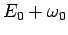occur at small momentum, implying a small effective coupling.

For a linear dispersion with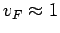or larger, the value ofwhere the first phonon threshold energy is reached is even smaller. Besides, the electronic density of states at small momentum, which is proportional to the number of relevant scatterings, is also much smaller then for a 1D tight-binding dispersion 4.3. These two conditions yield a very small effective coupling at the first phonon threshold. Consequently, even for large values ofas defined in Eq. 4.25, the polaron dispersion exhibits an extremely narrow gap, hardly discernible in our calculation.

The B model properties at larger momentum indicate an increase of the effective electron-phonon coupling with increasing the polaron momentum, due to the increase of the momentum of the relevant scattered phonons. For example, the most significant scattering at the two phonon threshold () are those for which. Ifis the value of the electron momentum for whichthe resulting values for the phonon momentumrelevant in the scattering are. The largersolution, i.e.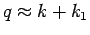(in Fig. 4.10-a), implies a larger effective coupling.

A similar analysis of the higher order self-energy diagrams leads to the same conclusion: The effective coupling in the B polaron model is increasing with momentum, and hence the small energy and momentum B polaron is characterized by a small effective coupling while the high energy and large momentum properties are determined by a large effective coupling. Moreover, at low energy the contribution of the crossing diagrams is comparable to the contribution of the crossing diagrams in a H model at small coupling. Thus, the SCBA solution is a good approximation for the low energy, small, polaron. Nevertheless, the large momentum properties are characterized by large effective couplings and hence the SCBA approximation is questionable in that region.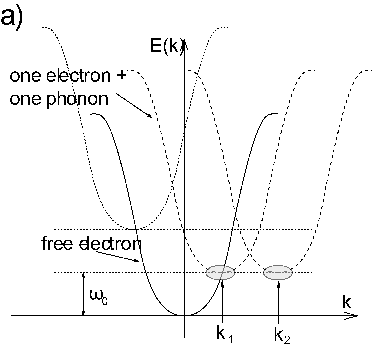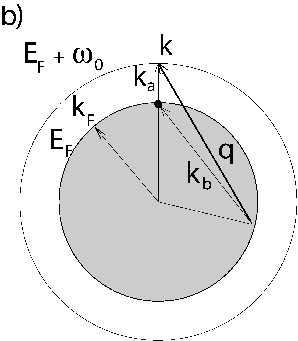The conclusions based on the analysis of self-energy diagrams discussed above implicitly assume that a smallimplies small, when the polaron momentumis small. However, as we have shown in the previous section, if the electron dispersion has low energy states separated by largethe above assumption is invalid. Thus the effective coupling at small energy is substantially larger and besides there is no significant increase of the effective coupling with polaron momentum or energy.

For example the strong dependence of the effective coupling on the momentum does not hold in a metallic system with a large Fermi surface. To see this, one can think of the Fermi surface as a degenerate ground state for the quasiparticle 4.4. Unlike the non-degenerate ground state case where the small energy scattering is restricted to small momentum, the relevant scatterings in the presence of a Fermi surface are restricted to small momentum plus a vector which connects two points on the Fermi surface (in Fig. 4.10 b)). Therefore scatterings with large momentum, for whichis large, are relevant. This makes the effective coupling to be large even at small energy. Because now at both low energy and high energy there are relevant large momentum scatterings the dependence of the effective coupling strength on the energy and momentum will be modest.

Although dimensionality plays an important role in determining the quantitative polaron properties (30,28), the main features of the B polaron are a consequence of strong momentum dependence of the bare electron-phonon coupling. Therefore the main conclusions of this study remain valid for 2D or 3D systems as long as the electron-phonon coupling has a similardependence (increases with).

The particularities of the B polaron discussed in this chapter might be relevant for the weakly doped cuprates characterized by very small Fermi pockets around the () points in the Brillouin Zone which makes the situation very similar to the one captured by the polaron model. The quasiparticles in this system have a four-fold degenerate ground state with the states separated byorvectors (20). The relevant phonon mode is believed to be the half-breathing one for which the electron-phonon coupling has a strongdependence, being small at smalland large at. If for some reasons the scattering between the () points is restricted or if its importance is small because of an additionaldependence in the bare electron-phonon couplingthe formed polarons will be characterized by a momentum and energy dependent effective coupling. Consequently, this implies interesting energy and temperature dependent properties such as a strongly temperature dependent quasiparticle photoemission linewidth (24). However, if the scattering between the () states is relevant, thedependence of the coupling will not be very important and presumably a Holstein like coupling can be used to describe the physics as well, analogous to the 1D case discussed in Fig. 4.7-f.Next: 4.5 Conclusions Up: 4. Spectral Properties of Previous: 4.3 Results
© Cyrill Slezak